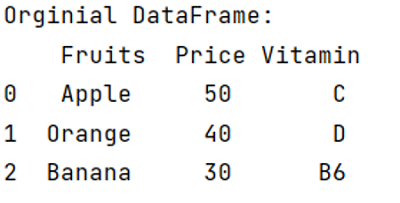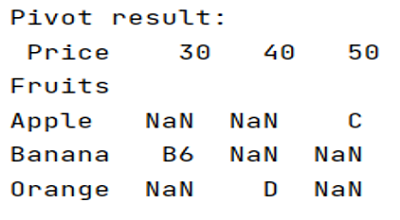# How to use pivot function in a pandas DataFrame?

Given a DataFrame, we have to use pivot function in it.
Submitted by Pranit Sharma, on May 04, 2022

Pandas is a special tool that allows us to perform complex manipulations of data effectively and efficiently. Inside pandas, we mostly deal with a dataset in the form of DataFrame. DataFrames are 2-dimensional data structures in pandas. DataFrames consist of rows, columns, and the data. A certain operation can be performed on DataFrames.

Many times, for a better understanding of datasets or to analyze the data according to our compatibility, we need to reorder or reshape the given DataFrame according to index and column values. DataFrame.pivot() helps us to achieve this task.

## pandas.DataFrame.pivot() Method

This method is used to reshape the given DataFrame according to index and column values. It is used when we have multiple items in a column, we can reshape the DataFrame in such a way that all the multiple values fall under one single index or row, similarly, we can convert these multiple values as columns. Suppose we have a column name ‘column’ having 3 repeating values (let p,q,r), if we pivot the DataFrame and define the columns = 'column', then the resulted pivot table will consist of 3 new columns named P, Q, R.

Syntax:

```df.pivot(index='col_name', columns='col_name', values='col_name')
```

Parameter(s):

• It takes the index as a parameter which is a column name and will be converted into a new row for the new DataFrame.
• It takes columns as a parameter which is a column name and will be converted into a new column for the new DataFrame.
• Also, it takes values as a parameter which is a column name and its values will be the final values of the new columns of the new DataFrame.

To work with pandas, we need to import pandas package first, below is the syntax:

```import pandas as pd
```

Let us understand with the help of an example:

```# Importing pandas package
import pandas as pd

# Creating dictionary
d = {
'Fruits':['Apple','Mango','Banana','Apple','Mango','Banana'],
'Price':[50,80,30,50,80,30],
'Vitamin':['C','C','B6','C','C','B6']
}

# Creating DataFrame
df = pd.DataFrame(d)

# Display original DataFrame
print("Original DataFrame:\n",df,"\n")
```

Output:We have created a DataFrame, now we will use the pivot() method to pivot this given DataFrame.

```# Pivot the DataFrame
result = df.pivot(index='Fruits', columns='Price', values='Vitamin')

# Display Pivot result
print("Pivot result:\n",result)
```

Output:Languages: » C » C++ » C++ STL » Java » Data Structure » C#.Net » Android » Kotlin » SQL
Web Technologies: » PHP » Python » JavaScript » CSS » Ajax » Node.js » Web programming/HTML
Solved programs: » C » C++ » DS » Java » C#
Aptitude que. & ans.: » C » C++ » Java » DBMS
Interview que. & ans.: » C » Embedded C » Java » SEO » HR
CS Subjects: » CS Basics » O.S. » Networks » DBMS » Embedded Systems » Cloud Computing
» Machine learning » CS Organizations » Linux » DOS
More: » Articles » Puzzles » News/Updates